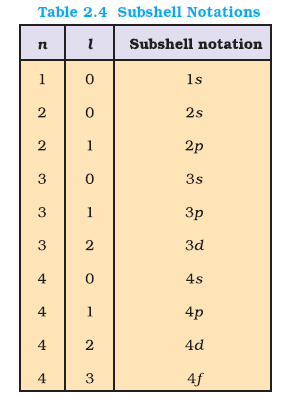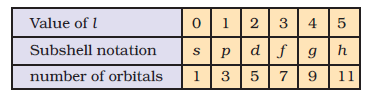Chemistry Quantum Mechanical Model of Atom, Quantum Numbers
Click for Only Video

### Topics to be covered :

● Quantum Mechanical Model of Atom .
● Quantum Mechanics.
● Important Feature of the Quantum Mechanical Model of Atom :
● Orbitals and Quantum Numbers :-
●Principle Quantum Numbers (n) :
● Azimuthal Quantum Number (l) :
● Magnetic Orbital Quantum Number (m_l) :
● Spin Quantum Number (m_s) :

### Quantum Mechanical Model of Atom :

Classic mechanics describe the motion of macroscopic objects but not of microscopic objects.

Note : This is due to the fact that classical mechanics does not consider the dual behaviour of matter and uncertainty principle.

### Quantum Mechanics :

Definition :- The branch of science which considers the dual behaviour of matter is called quantum mechanics.

Notes :- When quantum mechanics is applied to macroscopic objects, the result obtained is same as those obtained from the classical mechanics.

It was developed independently (1926) by Werner Heisenberg and Erwin Schrodinger.

Schrodinger developed the fundamental equation of quantum mechanics. This is expressed as : -

 hat H psi = E psi

where  hat H  is called Hamiltonian operator.

Note :- This expression is applicable to a system whose energy does not change with time.

The total energy of the system considers the kinetic energies of all the sub-atomic particles, attractive potential between electrons and nuclei and repulsive potential between electrons and nuclei individually.

Solution of this equation gives E and psi.

### Important Feature of the Quantum Mechanical Model of Atom :

Hydrogen Atom and the Schrodinger equation : -

(a) The solution of Schrodinger equation for H-atom gives the possible energy level the electron can occupy and the corresponding wave function (psi) of the electron associated with each energy level.

(b) These quantized energy level and corresponding wave function are characterised by quantum number which are as follow :-

(i) Principle Quantum number (n)

(ii) Azimuthal Quantum number (l)

(iii) Magnetic Quantum number (m_l)

(c) The wave function corresponding to a particular energy state gives all information about the electron.

(d) The value of wave function depends upon the coordinates of the electron in the atom.

(e) The wave function psi has no physical meaning .

(f) Wave function of the species having only one electron are called atomic orbitals. These wave functions are called one electron systems.

(g) The probability of finding an electron at a point within an atom prop | psi |^2  at that points.

(h) The quantum mechanical results explain all the aspect of H-atom spectrum.

Note : - Schrodinger equation cannot be solved exactly for a multi-electron atom. So for multi-electron system we use approximate method.

(i) Orbitals in atoms other than H-atom do not differ very much from the hydrogen atom. The principle difference is : -

(i) only nuclear charge increases and as a result orbitals get contracted.

(ii) For H-atom, energy of orbital prop n

(j) For multi-electron atoms, energy of orbital depends on (n and l).

### Orbitals and Quantum Numbers :-

(i) There are a large number of orbitals possible in an atom.

(ii) These orbitals are distinguised by Quantum Numbers.

(iii) Each orbital is designated by three Quantum Numbers n, l and m_l.

### Principle Quantum Numbers (n) :

(a) It can have any positive integer values i.e. n = 1,2,3,...

(b) It determines size and energy of the orbital.

(c) As n increases the size of orbital increases.
The energy of orbital increase with increase in value of n

(d) It also identifies the ''shell''

(e) The number of allowed orbitals = n^2

(f) All Orbitals belonging to a given value of n constitute a shell of atom and it is represented as

n \ \ \ \ \ \ \ \ \ \ \ \ \ \ 1 \ \ \ \ \ \ 2 \ \ \ \ \ 3 \ \ \ \ \ \ 4
text(shell) \ \ \ \ \ \ \ \ K \ \ \ \ L \ \ \ \ M \ \ \ \ N

Q 2632545432What is the total number of orbitals associated with the principal quantum number n = 3 ?Solution:

For n = 3, the possible values of l are 0, 1 and 2. Thus there is one 3s orbital (n = 3, l = 0 and m_l = 0);

there are three 3p orbitals (n = 3, l = 1 and m_l = –1, 0, +1);

there are five 3d orbitals (n = 3, l = 2 and m_l = –2, –1, 0, +1+, +2).

Therefore, the total number of orbitals is 1+3+5 = 9

The same value can also be obtained by using the relation; number of orbitals
= n^2, i.e. 3^2 = 9.

### Azimuthal Quantum Number (l) :(a) It is also known as orbital angular momentum or subsidiary quantum number.

(b) It defines 3D shape of the orbital.

(c) For a given value of n, l can have n values ranging from 0 to (n - 1) i.e. l = 0, 1, 2, 3 ......... ( n - 1)

e.g when n = 1 , l = 0

when n = 2 , l = 0, 1

(d) There can be one or more sub-shell or sub levels for a given shell.

(e) The number of sub-shell in a principal shell is equal to the value of n

e.g for n =1, number of sub-shell = 1
for n = 2, number of sub-shell = 2

(f) Sub-shells with different values of l are represented as : -

text(Value for) \ \ l : \ \ \ \ \ \ \ \ \ \ \ \ \ \ \ \ \ \ \ \ \ \ \ \ \ 0 \ \ \ \ 1 \ \ \ \ 2 \ \ \ \ \ 3 \ \ \ \ 4 \ \ \ \ 5
text(Notation for sub shell ) : \ \ s \ \ \ \ p \ \ \ \ d \ \ \ \ \ f \ \ \ \ g \ \ \ \ h

See table 2.4

Q 2652545434Using s, p, d, f notations, describe the orbital with the following quantum numbers

(a) n = 2, l = 1, (b) n = 4, l = 0, (c) n = 5, l = 3, (d) n = 3, l = 2Solution:

\ \ \ \ \ \ \ n \ \ l \ \ \ text(orbital)
(a) \ \ \ \ \ \ \ \ \ \ \ \ \ 2 \ \ \ 1 \ \ \ \ 2p
(b) \ \ \ \ \ \ \ \ \ \ \ \ \ 4 \ \ \ \0 \ \ \ \ 4s
(c) \ \ \ \ \ \ \ \ \ \ \ \ \ 5 \ \ \ \ 3 \ \ \ \ 5f
(d) \ \ \ \ \ \ \ \ \ \ \ \ \ 3 \ \ \ \ 2 \ \ \ \ 3d

### Magnetic Orbital Quantum Number (m_l) :(a) It gives details about the spatial orientation of the orbital in the space.

(b) for any value of sub shell i.e. l , there can be (2l + 1) value of m_l. These values are given as :

m_l = - l , - (l - 1) , - ( l - 2) ....... 0,1,2, ....... ( l- 2),(l - 1) , l

e.g for l = 0, m_l = ( 2 xx 0 + 1) = 1 (one s-orbital)
for l = 1, m_l = ( 2 xx 1 + 1) = 3 (three p-orbital)
and m_l = -1, 0, 1

(c) The relation between the sub-shell and the number of orbitals associated with it is given in the Table.
Q 1935801762An electron is in one of the 3d orbitals. Give the possible values of n, l and m_l for this electron
Class 11 Exercise 2 Q.No. 25Solution:

For 3d orbital, n=3,l=2. For l=2,m =-2-1,
0, +1+2.

### Spin Quantum Number (m_s) :

The above three quantum numbers were unable to explain the line spectra observed in the case of multi-electron atoms. This suggests the presences of a few more energy levels.

George Uhlenbeck and Samuel Goudsmit proposed the presence of the fourth quantum number known as the electron spin quantum numbers (m_s).

An electron spins around its own axis. So, it has intrinsic spin angular quantum number. Since spin angular momentum is vector quantity it can have two orientations are distinguished relative to its axis and these two orientations are distintinguised by spin quantum number (m_s). m_s can have values of  + 1//2 or  - 1//2. These are called the two spin states of the electron and represented as :-

uparrow ( spin up) and downarrow

An orbital can not hold more then two electrons and these two electron have opposite spins.
Q 1965312265Explain, giving reasons, which of the following
sets of quantum numbers are not possible.
(a) n=0, l=0, m_1=0, m_s=+ 1/2

(b) n=1, l=0, m_1=0, m_s= -1/2

(c) n=1, l=1, m_1=0, m_s=+1/2

(d) n =2, l = 1, m_1=0, m_s=-1/2

(e)  n=3, l=3, m_1=-3, m_5 =+1/2

(f) n=3, l= 1, m_1=0, m_s=+ 1/2

Class 11 Exercise 2 Q.No. 30Solution:

(a) Not possible because n can never be equal to, zero.
(b) Possible
(c) Not possible
(d) Possible
(e) Not possible because when n = 3, l ne 3 as
value of l varies from 0 to n- 1.
(f) Possible